# IV Drug Administration Drug Calculations November 2007 Drug

• Slides: 28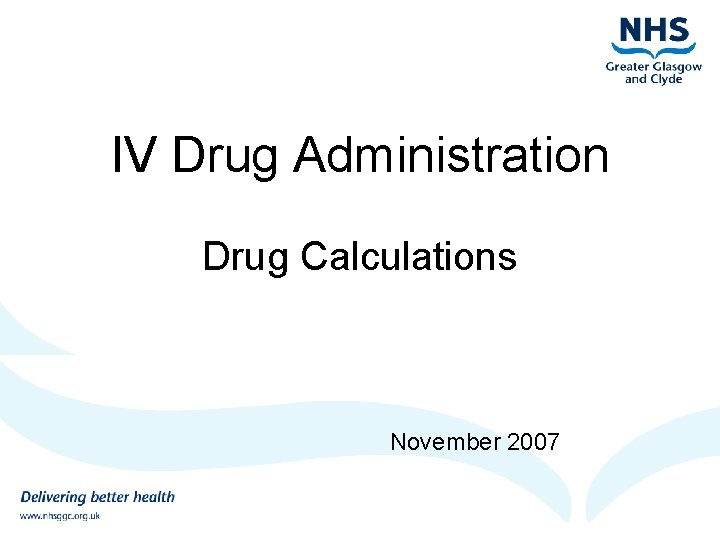IV Drug Administration Drug Calculations November 2007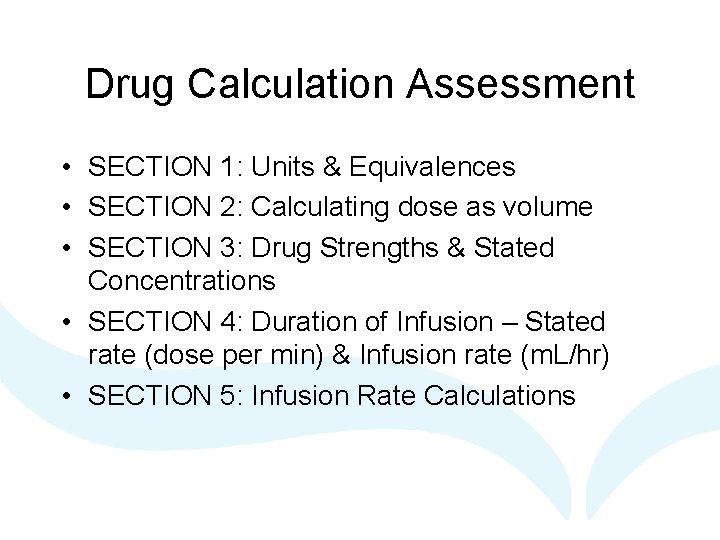Drug Calculation Assessment • SECTION 1: Units & Equivalences • SECTION 2: Calculating dose as volume • SECTION 3: Drug Strengths & Stated Concentrations • SECTION 4: Duration of Infusion – Stated rate (dose per min) & Infusion rate (m. L/hr) • SECTION 5: Infusion Rate Calculations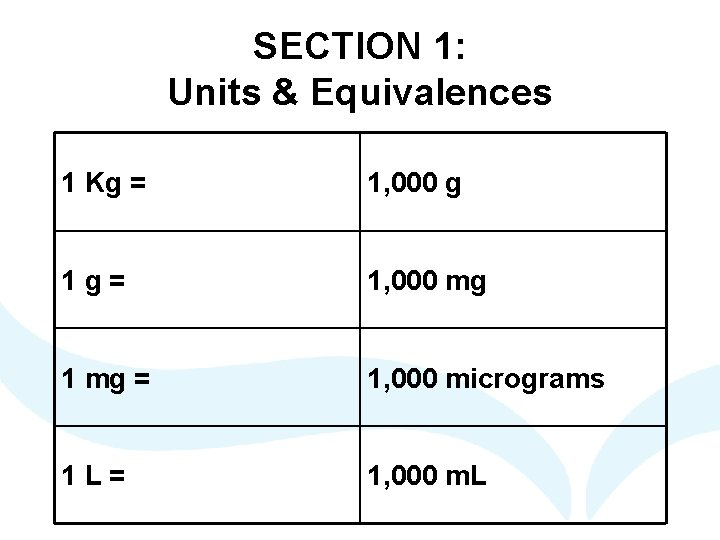SECTION 1: Units & Equivalences 1 Kg = 1, 000 g 1 g= 1, 000 mg 1 mg = 1, 000 micrograms 1 L= 1, 000 m. LConverting from One Unit to Another • To convert from a larger unit to a smaller unit, multiply by 1000 or move the decimal point three places to the right • To convert from a smaller unit to a larger unit, divide by 1000 or move the decimal point three places to the leftUnits & Equivalences Convert 0. 25 mg into micrograms Convert 600 micrograms to mg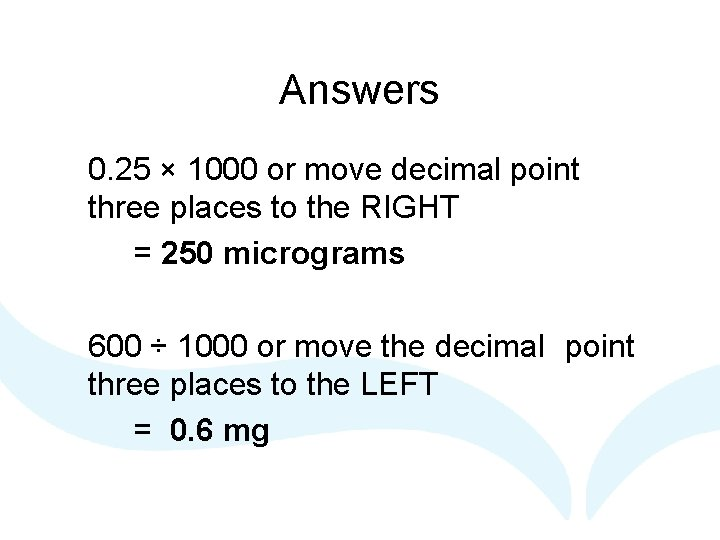Answers 0. 25 × 1000 or move decimal point three places to the RIGHT = 250 micrograms 600 ÷ 1000 or move the decimal point three places to the LEFT = 0. 6 mg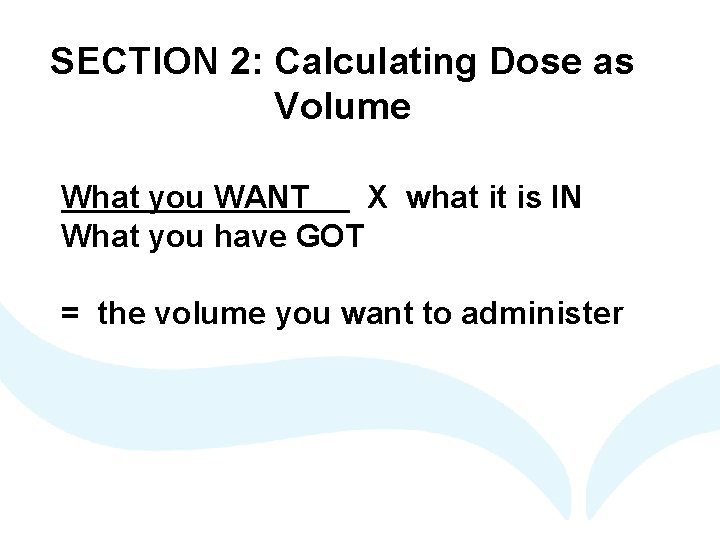SECTION 2: Calculating Dose as Volume What you WANT X what it is IN What you have GOT = the volume you want to administerA patient is prescribed 200 mg of Furosemide I. V. The ampoules available contain 250 mg in 10 m. L. What volume containing the drug do you need to administer? Time Furosemide 0700 -0900 1200 -1400 Dose 200 mg Route Start Date IV 18. 05. 07 End Date What it is IN 1600 -1800 2200 -2400 Other Time Signature of Doctor Furosemide 250 mg/10 m. L P. Rescriber What we WANT What we have GOT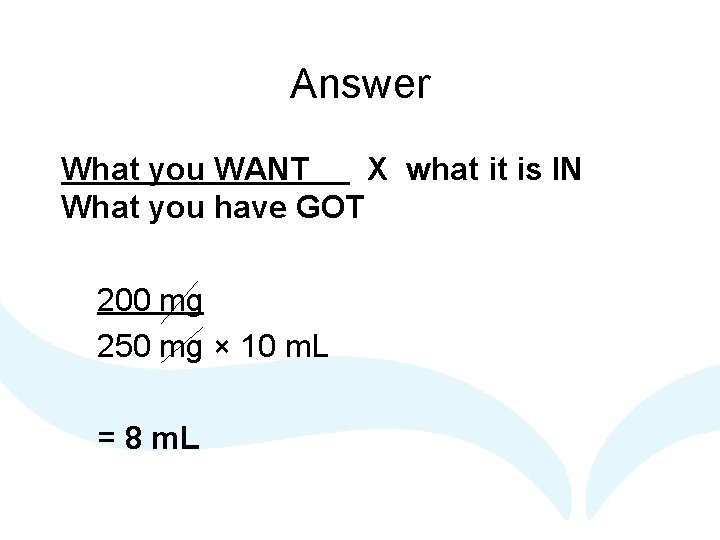Answer What you WANT X what it is IN What you have GOT 200 mg 250 mg × 10 m. L = 8 m. LSECTION 3: Drug Strengths & Stated Concentrations What you HAVE (mg) CONCENTRATION (mg/m. L) = How much fluid is required (Volume)A patient is prescribed 250 mg of Aciclovir I. V. Aciclovir has to be initially reconstituted with water for injection, then added to a bag of compatible infusion fluid, giving a final concentration of 5 mg/m. L. How much infusion fluid should be used dilute the reconstituted Aciclovir to achieve this final concentration? Time Aciclovir 0700 -0900 1200 -1400 Dose 250 mg Route Start Date IV 18. 05. 07 End Date 1600 -1800 2200 -2400 Other Time What we HAVE Signature of Doctor Additional Comments P. Rescriber Final Concentration 5 mg/m. L CONCENTRATION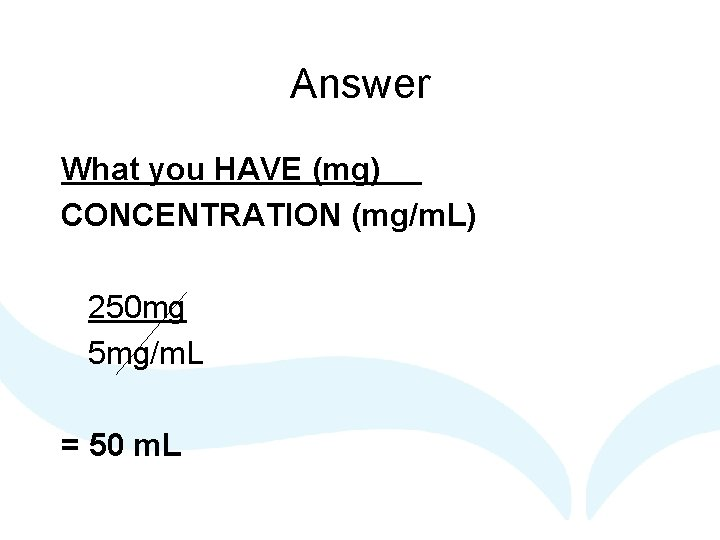Answer What you HAVE (mg) CONCENTRATION (mg/m. L) 250 mg 5 mg/m. L = 50 m. L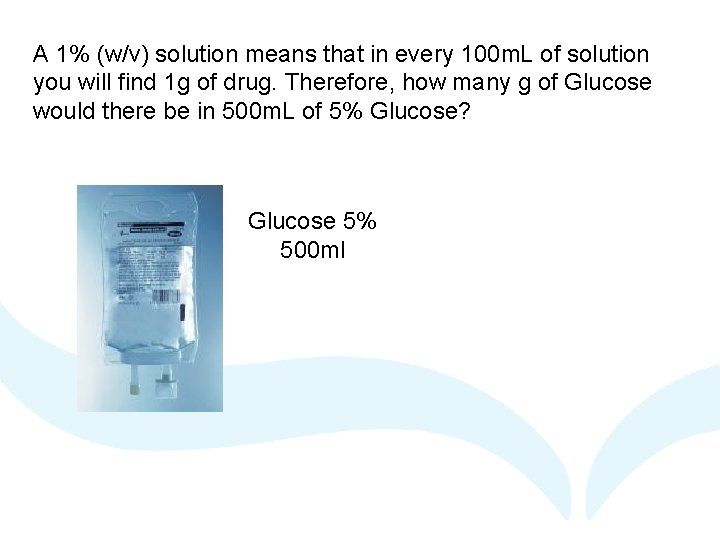A 1% (w/v) solution means that in every 100 m. L of solution you will find 1 g of drug. Therefore, how many g of Glucose would there be in 500 m. L of 5% Glucose? Glucose 5% 500 ml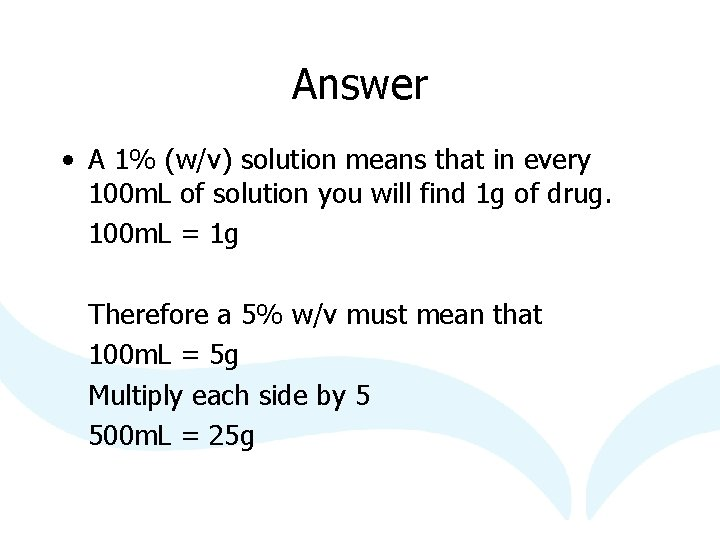Answer • A 1% (w/v) solution means that in every 100 m. L of solution you will find 1 g of drug. 100 m. L = 1 g Therefore a 5% w/v must mean that 100 m. L = 5 g Multiply each side by 5 500 m. L = 25 g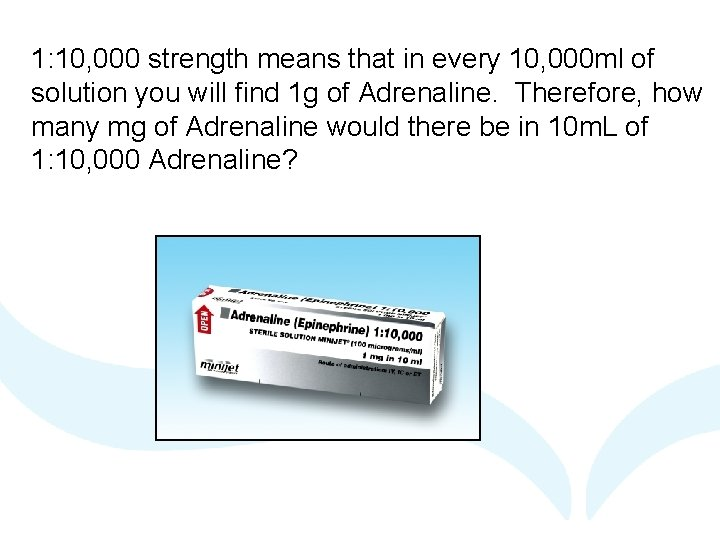1: 10, 000 strength means that in every 10, 000 ml of solution you will find 1 g of Adrenaline. Therefore, how many mg of Adrenaline would there be in 10 m. L of 1: 10, 000 Adrenaline?Answer • 1: 10, 000 means that 1 g of adrenaline is dissolved in 10, 000 m. L. 1 g = 10, 000 m. L 1000 mg = 10, 000 m. L Divide both sides by 1000 1 mg = 10 m. LSECTION 4: Duration of Infusion Stated rate (dose per min) Infusion rate (m. L/hr) Stated Rate – dose per min Dose (mg) = Time Rate (mg/min)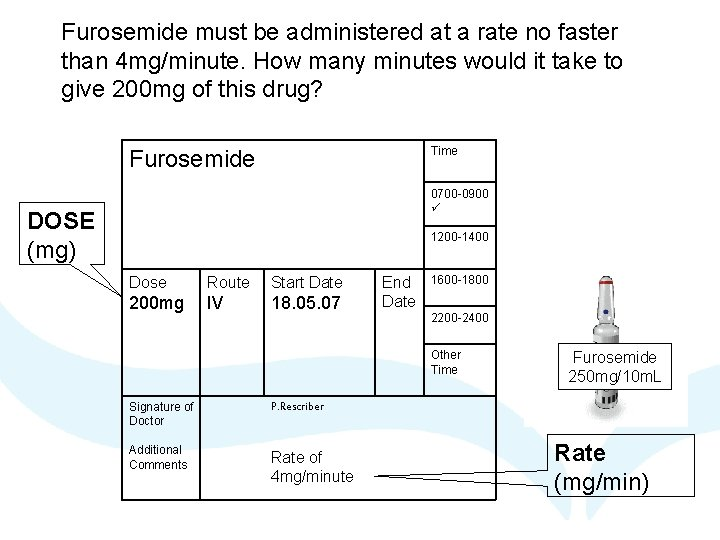Furosemide must be administered at a rate no faster than 4 mg/minute. How many minutes would it take to give 200 mg of this drug? Time Furosemide 0700 -0900 DOSE (mg) 1200 -1400 Dose Route Start Date 200 mg IV 18. 05. 07 End Date 1600 -1800 2200 -2400 Other Time Signature of Doctor P. Rescriber Additional Comments Rate of 4 mg/minute Furosemide 250 mg/10 m. L Rate (mg/min)Answer Dose (mg) = Time Rate (mg/min) 200 mg 4 mg/min = 50 minutes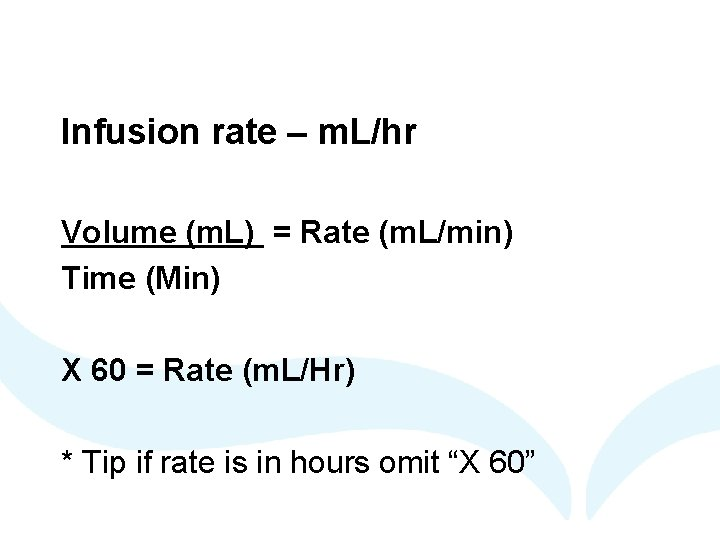Infusion rate – m. L/hr Volume (m. L) = Rate (m. L/min) Time (Min) X 60 = Rate (m. L/Hr) * Tip if rate is in hours omit “X 60”Omeprazole (Losec ®) 40 mg is diluted in 100 m. L of compatible infusion fluid. This infusion has to be given over 20 minutes. What rate should you set the infusion pump at, in ml/hr, to deliver this dose? Omeprazole 40 mg/100 m. L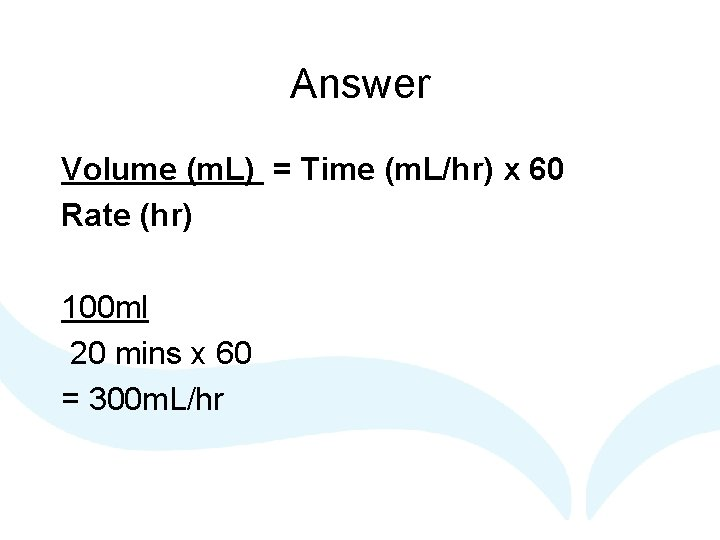Answer Volume (m. L) = Time (m. L/hr) x 60 Rate (hr) 100 ml 20 mins x 60 = 300 m. L/hr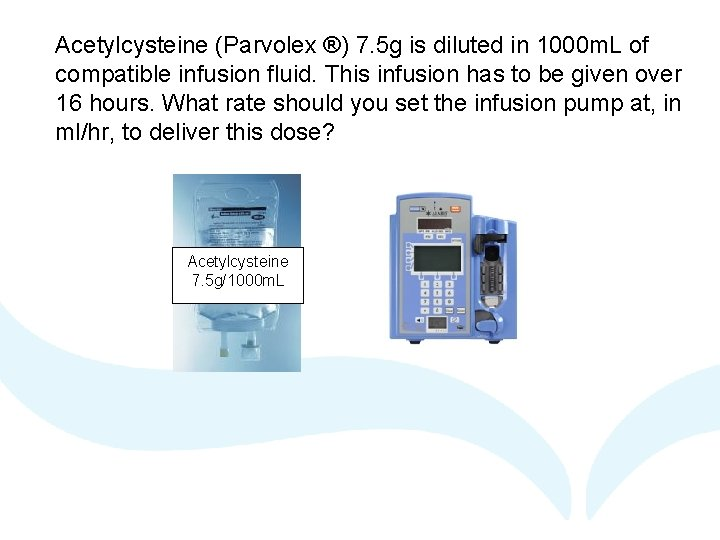Acetylcysteine (Parvolex ®) 7. 5 g is diluted in 1000 m. L of compatible infusion fluid. This infusion has to be given over 16 hours. What rate should you set the infusion pump at, in ml/hr, to deliver this dose? Acetylcysteine 7. 5 g/1000 m. LAnswer Dose (m. L) = Time (m. L/hr) Rate (hr) 1000 16 = 62. 5 m. L/hr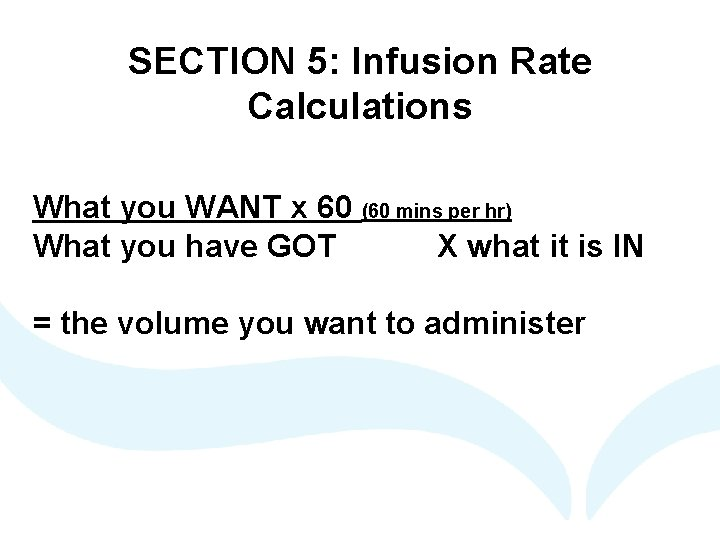SECTION 5: Infusion Rate Calculations What you WANT x 60 (60 mins per hr) What you have GOT X what it is IN = the volume you want to administerYou are required to give a patient Glyceryl Trinitrate as a continuous I. V infusion at 25 micrograms/minute. You have prepared a 50 mg in 50 m. L infusion. What rate should you set the infusion pump at, in ml/hr, to deliver this dose? What we Time Glyceryl Trinitrate 07000900 What we WANT 12001400 Dose Route 25 micrograms/ IV minute 60 mins per hr Start Date 18. 05. 07 End Date 16001800 have GOT Glyceryl Trinitrate 50 mg/50 m. L 22002400 Other Time Signature of Doctor P. Rescriber Additional Comments Continuous Infusion What its IN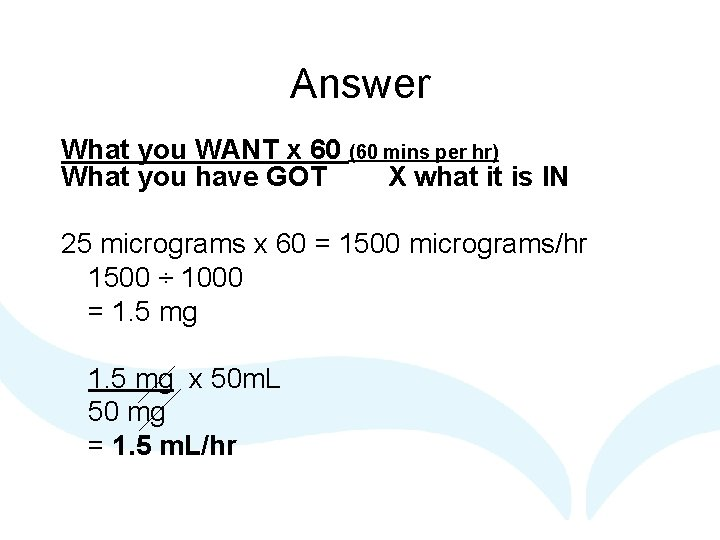Answer What you WANT x 60 (60 mins per hr) What you have GOT X what it is IN 25 micrograms x 60 = 1500 micrograms/hr 1500 ÷ 1000 = 1. 5 mg x 50 m. L 50 mg = 1. 5 m. L/hr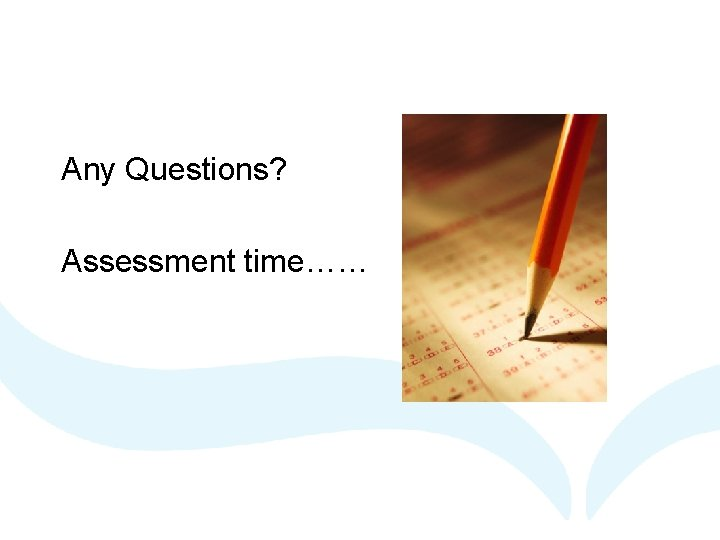Any Questions? Assessment time……